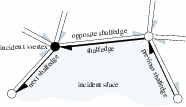## CGAL::Nef_polyhedron_S2<Traits>::SHalfedge

### Definition

A shalfedge is a great arc on a sphere map. The figure below depicts the relationship between a shalfedge and its incident shalfedges, svertices, and sfaces on a sphere map. A shalfedge is an oriented sedge between two svertices. It is always paired with a shalfedge pointing in the opposite direction. The twin() member function returns this shalfedge of opposite orientation.Figure 17.2:  Incidences of an SHalfedge

The snext() member function points to the successor shalfedge around this sface while the sprev() member function points to the preceding shalfedge. An successive assignments of the form se = se->snext() cycles counterclockwise around the sface (or hole).

Similarly, the successive assignments of the form se = se->snext()->twin() cycle clockwise around the svertex and traverse all halfedges incident to this svertex. The assignment se = se->cyclic_adj_succ() can be used as a shortcut.

A const circulator is provided for each of the two circular orders. The circulators are bidirectional and assignable to SHalfedge_const_handle.

#include <CGAL/Nef_polyhedron_S2.h>

### Types

The following types are the same as in Nef_polyhedron_S2<Traits>.

 Nef_polyhedron_S2::SHalfedge::Mark type of mark. Nef_polyhedron_S2::SHalfedge::Sphere_circle sphere circle type stored in SHalfedge. Nef_polyhedron_S2::SHalfedge::SVertex_const_handle const handle to SVertex. Nef_polyhedron_S2::SHalfedge::SHalfedge_const_handle const handle to SHalfedge. Nef_polyhedron_S2::SHalfedge::SFace_const_handle const handle to SFace.

### Creation

There is no need for a user to create a SHalfedge explicitly. The class Nef_polyhedron_S2<Traits> manages the needed shalfedges internally.

### Operations

 Mark se.mark () the mark of se . Sphere_circle se.circle () the sphere circle of se . SHalfedge_const_handle se.twin () the twin of se . SVertex_const_handle se.source () the source svertex of se . SVertex_const_handle se.target () equals twin()->source(). SHalfedge_const_handle se.sprev () the SHalfedge previous to se in a sface cycle. SHalfedge_const_handle se.snext () the next SHalfedge of se in a sface cycle. SHalfedge_const_handle se.cyclic_adj_pred () the edge before se in the cyclic ordered adjacency list of source(). SHalfedge_const_handle se.cyclic_adj_succ () the edge after se in the cyclic ordered adjacency list of source(). SFace_const_handle se.incident_sface () the incident sface of se .